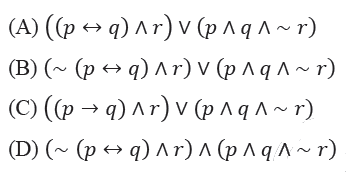GATE | GATE-CS-2014-(Set-1) | Question 63

• Difficulty Level : Medium
• Last Updated : 11 Sep, 2017

Which one of the following propositional logic formulas is TRUE when exactly two of p, q, and r are TRUE?(A) A
(B) B
(C) C
(D) D

Explanation: Draw the truth table of three variables and output will be 1 (true) only when exactly two variables are 1 (true) else output will be 0 (false).
Therefore,

p    q     r   Output (f)
0    0    0    0
0    0    1    0
0    1    0    0
0    1    1    1
1    0    0    0
1    0    1    1
1    1    0    1
1    1    1    0

f = ( ~p ∧ q ∧ r) ∨ (p ∧ ~q ∧ r) ∨ (p ∧ q ∧ ~r)
f = {( ~p ∧ q ) ∨ (p ∧ ~q )} ∧ r  ∨ (p ∧ q ∧ ~r)
f = {(p ⊕ q )} ∧ r  ∨ (p ∧ q ∧ ~r)
f = {~ (p ↔ q )} ∧ r  ∨ (p ∧ q ∧ ~r)

Hence, option (b) is true.

Please comment below if you find anything wrong in the above post.

Quiz of this Question

My Personal Notes arrow_drop_up